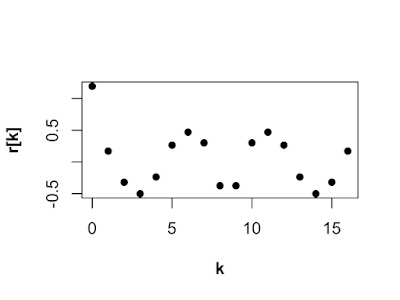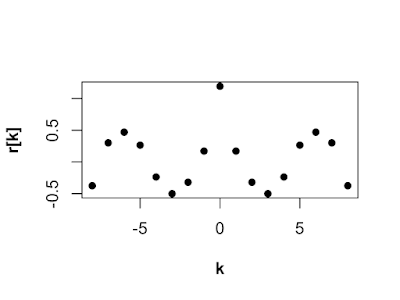## Monday, May 27, 2019

### Book Review: Data Analysis with Open Source Tools

This book gives a very good overview of the kinds of things data scientists do and the tools they use.  All the concepts are illustrated with hands on programing examples you can follow along with.

I think this book would be very good for two groups of people. First, someone working in a technical field who wants to know what data science is all about. This would give them a good idea of the skills they will need and the type of work they may do as a data scientist.

The second group that would benefit from this book are people engaged in some field of data science and who are looking for a broad overview of the field. Since many people came to data science from other fields and learned hands on they may have trouble seeing the forest for the trees.

This is not a pop science book. If you looking for an armchairs introduction to the wonderful world of data science, this is not the book for you. If you want to learn by doing and know a bit of math and have some familiarity with programing, then this is the book for you.

https://www.amazon.com/Data-Analysis-Source-Tools-Hands/dp/0596802358

## Sunday, May 26, 2019

### Mirror image data with MATLAB

A sequence is even if x[midpoint-n] = x[midpoint+n] for some midpoint and for all n. There are many cases where it is handy to have a even sequence. For Instance, the Fourier transform of an even sequence is real and it is handy to have a real function in the transform domain.

Below is the some MATLAB code that will make a sequence even by appending a mirror image section. The function will mirror image one, two and three dimensional data.

function DataMirrorImage = MirrorImageData(Data)
% This function mirror images one, two and three dimensional data in all
% in one, two or three dimensions.
%
% INPUT:
% Data3D................The data to be mirror imaged.
%
% OUTPUT:
% DataMirrorImage.....The mirror image data.

% Get the dimensions of the data.
[xLen, yLen, zLen] = size(Data);

% Mirror image the data in 1D or 2D.
for zNdx = 1:zLen
Data2D = squeeze(Data(:,:,zNdx));
Data2D = [ Data2D fliplr(Data2D(:,2:yLen))];
Data2DMirrorImage = [ Data2D; flipud(Data2D(2:xLen,:))];
DataMirrorImage(:,:,zNdx) = Data2DMirrorImage;
end

% If data is 3D , mirror image the data in 3D.
if zLen > 1
Data3DMirrorImage(:,:,1:zLen) = DataMirrorImage;
Data3DMirrorImage(:,:,(zLen+1):(2*zLen-1)) = flipdim(DataMirrorImage(:,:,2:zLen),3);
DataMirrorImage = Data3DMirrorImage;
end

Example:

>> x = randn(1,9)
x =
1.4172    0.6715   -1.2075    0.7172    1.6302    0.4889    1.0347    0.7269   -0.3034
>> xMi = MirrorImageData(x)
xMi =
Columns 1 through 10
1.4172    0.6715   -1.2075    0.7172    1.6302    0.4889    1.0347    0.7269   -0.3034   -0.3034
Columns 11 through 17
0.7269    1.0347    0.4889    1.6302    0.7172   -1.2075    0.6715

>> x = randn(9);
>> imagesc(x)

>> imagesc(MirrorImageData(x))

## Saturday, May 25, 2019

### 'Semi-infinite' Sounds like a lot!

Looks like we'll be making computers for a while. https://www.independent.co.uk/environment/rare-earth-metals-japan-semi-infinite-ocean-mobile-phones-electric-cars-a8301966.html

## Friday, May 24, 2019

### Random Autocorrelation Sequences R version

Random Autocorrelation Sequences R version

### What is an autocorrelation sequence?

Autocorrelation sequences (ACSs) are super common when doing anything in probability and statistics. Autocorrelation is a sequence of measurements of how similar a sequence is to it self. In math the autocorrelation sequence r[k] is

r[k] = E[x[n]x[n+k]] for k={0,1,...N-1},

where N is the number of data samples, E is the expected value, x[n] is a data sample and k is the lag. The lag is the separation in samples.

### Why make a random autocorrelation sequence?

When testing an algorithm or conducting simulations it is often useful to use a random ACS. Generating random a random ACS can be difficult because they have a lot of special properties and if you select a sequence at random, the chance it is a valid ACS is small.

### Trick to making a random autocorrelation sequence

We can use the following property of ACSs to make generating random ACSs easy. The ACS and the power spectral density (PSD) are Fourier transform (FT) pairs. For our purpose here, a PSD is just a function that is positive everywhere. "FT pair" means the FT of an ACS is a PSD and the inverse FT of a PSD is an ACS.

So we can generate a random ACS using the following steps. First, generate a random sequence. Second, square each element, so the sequence is positive. Finally, find the inverse FT of the squared sequence.

### The R code that produces a random ACS

The ACS could be any size, but in this case we want a 9 element sequence.

N <- 9
PSD <- rnorm(N)^2
ACS <- fft(PSD,inverse = TRUE)

The line below outputs the ACS and as you can see it is a complex sequence.

ACS

 0.6183715+0.0000000i -0.1375219+0.1960568i -0.1672163-0.2084656i 0.2199730-0.0977208i
 -0.0281983+0.2615475i -0.0281983-0.2615475i 0.2199730+0.0977208i -0.1672163+0.2084656i
 -0.1375219-0.1960568i

### What if I want a real ACS

If you want a real ACS then the PSD has to be even. So, let's make the sequence even!

PSDeven <- c(PSD,PSD[N:2])
PSDeven

 0.39244438 0.03372487 0.69827518 2.54492084 0.10857537 0.67316837 0.23758708 0.54512337
 0.33152416 0.33152416 0.54512337 0.23758708 0.67316837 0.10857537 2.54492084 0.69827518
 0.03372487

Notice the ACS is still complex. Numerical error causes some imaginary dust we need to clean up.

ACS <- fft(PSDeven,inverse = TRUE)/N
ACS

 1.1931381+0i 0.1714080+0i -0.3200109+0i -0.5007558+0i -0.2372697+0i 0.2647028+0i
 0.4700409+0i 0.3009945+0i -0.3750372+0i -0.3750372+0i 0.3009945+0i 0.4700409+0i
 0.2647028+0i -0.2372697+0i -0.5007558+0i -0.3200109+0i 0.1714080+0i

Clean up the small imaginary part with Re() and now we are ready to plot.

ACS <- Re(ACS)

### Plot of ACS

The figure below is a plot of the ACS from lag k = 0 to 16. In textbooks the ACS would have been plotted from k=-8 to 8, with r in the center.This is the plot of the ACS in the textbook style. Notice, the lag at 0 r is positive and larger than the other lags, a standard property of ACSs. All is well!## Thursday, May 23, 2019

This is a generated video of someone talking made from one  image!!

https://youtu.be/p1b5aiTrGzY?t=47

## Wednesday, May 22, 2019

### Random Autocorrelation Sequences MATLAB version

Autocorrelation sequences (ACSs) are commonly used in a variety of fields. When testing an algorithm or conduction simulations it is sometimes useful to use a random ACS. Generating random a random ACS can be difficult because they have a lot of special properties and if you select a sequence at random, the chance it is a valid ACS is small.

We can use the following property of ACSs to make generating random ACSs easy. The ACS and the power spectral density (PSD) are Fourier transform (FT) pairs. For our purpose here, a PSD is just a function that is positive everywhere. "FT pair" means the FT of an ACS is a PSD and the invers FT of a PSD is an ACS.

So we can generate a random ACS using the following steps. First, generate a random sequence. Second, square each element. Finally, find the inverse FT of the squared sequence.

The matlab code below does just that.
N = 9;
PSD = randn(1,N).^2;
ACS = ifft(PSD);
ACS
ACS =
1.0022 + 0.0000i  -0.0828 + 0.1658i   0.1847 - 0.0446i   0.0978 + 0.1778i   0.3034 - 0.3709i   0.3034 + 0.3709i   0.0978 - 0.1778i   0.1847 + 0.0446i  -0.0828 - 0.1658i

## Tuesday, May 21, 2019

### Testing vs. Developing

My favorite probability mistake is mistaking developing a hypothesis for testing a hypothesis*. This one is easy to make and it make by lots of people. Smart people and dumb people, people in a variety of fields: finance, self-help**, and history. This basic mistake has two parts. First, look at some data and make a hypothesis: a trend, a connection, a pattern. Second, claim the data "proves" the hypothesis.

Finding something interesting in a data set is good! The next step should be to get more data and see if it has the same interesting thing. You can't test a hypothesis with the same data set that you used to develop the hypothesis!

You could get some data and fit a model to it. The hypothesis would be, "I think the model predicts future data".  Maybe the model predicts future data, but maybe not! The only way to know is if you get more data (this means you have to collect or find new data) then test your model with the new data. If the model fits the new data and the old data, maybe you have something.

*   A hypothesis is a guess you plan to test.
** This is super common in self-help books. Every self-help book “I talked to 20 rich guys and they did this thing, so you should do it to!”